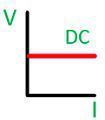# Difference Between AC & DC Voltage

The major difference between the AC and DC voltage is that in AC voltage the polarity of the wave changes with the time whereas the polarity of the DC voltage always remains same. The other differences between the AC and DC voltage are shown below in the comparison chart.

## Comparison Chart

Basis for Comparison AC Voltage DC Voltage
DefinitionThe AC voltage is the force that derive the alternating current between the two points. The DC Voltage induces the direct current between the two points.
Symbolic RepresentationFrequency Depends on country. Zero
Power Factor Lies between 0 to 1. 0
Polarity Changes Remain Constant
DirectionVary Remain same
Obtained From Generator Cell or Battery
Efficiency High Low
Passive Parameter ImpedanceResistance
Amplitude HaveDon't Have
ConversionBy using inverter. By using rectifier.
Transformer Requires for transmission.Not requires.
Phase and Neutral HaveDon't Have

### Definition of AC Voltage

The voltage which causes the alternating current is known as the AC voltage. The alternating current induces in the coil when the current carrying conductor rotates in the magnetic field. The conductor when rotates cuts the magnetic flux and the variation of the flux induces the alternating voltage in the conductor.

### Definition of DC Voltage

The dc voltages induce the direct current. The waves only in one direction and the magnitude of the voltage always remain constant. The generation of the DC voltage is quite simple and easy. The voltage induces by rotating the coil in the field of the magnet. The coil consists the split ring and commutator which converts the alternating voltage into the direct voltage.

## Key Differences Between AC and DC Voltage

1. The voltage which causes the alternating current is known as the alternating voltage. The DC voltage produces the direct current.
2. The frequency of the AC voltage depends on the country (mostly 50 and 60 Hz are used). Whereas, the frequency of DC voltage becomes zero.
3. The power factor for AC voltage lies between 0 to 1. And the power factor of the DC voltages always remains 1.
4. The polarity of the AC voltage always varies with time, and the polarity of the DC voltage always remain constant.
5. The AC voltage is unidirectional, and the DC voltage is bidirectional.
6. The generator generates the AC voltage, and the DC voltage is obtained from the cell or battery.
7. The efficiency of AC voltage is high as compared to the DC voltage.
8. The impedance is the passive parameter of the AC voltage, and for DC it is resistance. The impedance means the opposition offered by the voltage to the flow of current.
9. The AC voltage has amplitude whereas the DC voltage doesn’t have amplitude. The term amplitude means the maximum distance covered by the oscillation and the vibrating body.
10. The inverter converts the direct current into the alternating current. Whereas, the rectifier converts the alternating current into the direct current.
11. The transformer is essentials for AC transmission while it is not used in DC transmission.
12. The ac voltage has phase and neutral whereas the DC voltage neither requires phase nor neutral.
13. The main advantage of the AC voltage is that it is easily measured. The advantage of the DC voltage is that the voltage can easily be amplified. The amplification is the process through which the strength of the signal increases.

### Relation Between AC and DC Voltage

AC Volts Χ 1.414 = DC Volts